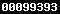### EKSPERIMENTASI MODEL PEMBELAJARAN PROBLEM BASED LEARNING (PBL), DISCOVERY LEARNING (DL), DAN PROBLEM POSSING (PP) DITINJAU DARI KECERDASAN MAJEMUK SISWA PADA MATERI KUBUS DAN BALOK SMP NEGERI KABUPATEN DEMAK

Shanti Indah Lestari, Budiyono Budiyono, Isnandar Slamet

#### Sari

Abstract: The objective of this research is to investigate the effect of learning models on the learning achievement in mathematics viewed from the multiple intelligences. The learning models compared were the PBL, DL, and PP models. Its population was all of the students in Grade VIII of State Junior Secondary Schools of Demak. The samples of research were taken by using the stratified cluster random sampling technique. The proposed hypotheses of research were tested by using the two-way analysis of variance with unbalanced cells. The results of research are as follows. 1) The students instructed with the PBL model have a better learning achievement in Mathematics than those instructed with the DL and PP models, and the students instructed with the DL model results in a better learning achievement in Mathematics than those instructed with the PP model. 2) The students with the logical-mathematical intelligence have a better learning achievement in Mathematics than those with the visual and interpersonal intelligences, and the students with the visual intelligence have a better learning achievement in Mathematics than those with the interpersonal intelligence. 3) In the students with the logical-mathematical and visual intelligences, the PBL model results in the same learning achievement in Mathematics as the DL, the DL model results in the same learning achievement in Mathematics as the PP model, and the PBL model results in the same learning achievement in Mathematics as the PP model. In the students with the interpersonal intelligence, the PBL model results in the same learning achievement in Mathematics as the DL, the DL model results in the same learning achievement in Mathematics as the PP model, but the PBL model results in a better learning achievement in Mathematics than the PP model. 4) In the PBL, DL , and PP models, the students with the logical-mathematical intelligence have the same learning achievement in Mathematics as those with the visual intelligence, the students with the visual intelligence have the same learning achievement in Mathematics as those with the interpersonal intelligence, and the students with the logical-mathematical intelligence have the same learning achievement in Mathematics as those with the interpersonal intelligence.

Keywords: Problem Based Learning (PBL), Discovery Learning (DL), Problem Possing (PP) and Multiple Intelligences.

PDF

### Refbacks

• Saat ini tidak ada refbacks.View My Stats